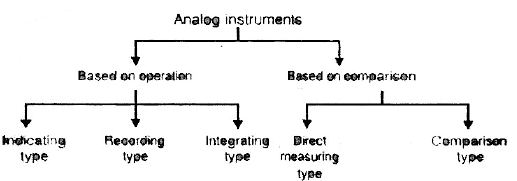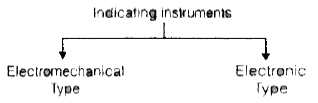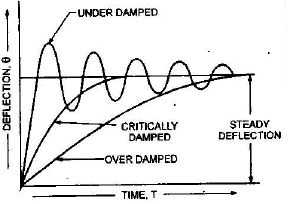Courses

# Chapter 3 Electromechanical Indicating Type Instruments(Part - 1) - Notes, Electrical Measurement Electrical Engineering (EE) Notes | EduRev

## Electrical Engineering (EE) : Chapter 3 Electromechanical Indicating Type Instruments(Part - 1) - Notes, Electrical Measurement Electrical Engineering (EE) Notes | EduRev

The document Chapter 3 Electromechanical Indicating Type Instruments(Part - 1) - Notes, Electrical Measurement Electrical Engineering (EE) Notes | EduRev is a part of the Electrical Engineering (EE) Course Electrical Engineering SSC JE (Technical).
All you need of Electrical Engineering (EE) at this link: Electrical Engineering (EE)Indicating instruments:

• Those instruments which indicate the magnitude of a quantity being measured.
• They generally make use of a dial and a pointer for this purpose.
• Example: Ordinary voltmeters, ammeters and wattmeters⇒ Electronic types of instruments are manufactured by addition of electronic circuits to electromagnetic indicators in order to increase the sensitivity and input impedance.

Recording Instruments:  It gives a continuous record of quantity being measured over a certain period of time.

Example : Reorders.

Integrating Instruments:  Ampere hour meter and watt hour meter (Energy meter) are the examples of this type.

Direct Measuring Instruments:  Ammeters, voltmeters, wattmeters and energy meters are the example of this type.

Comparison Instruments:  AC and DC bridges, potentiometer are the examples of this type.

Types of operating forces:

• Deflecting force
• Controlling force
• Damping force

Deflection force

• It is responsible for deflection in moving system.
• It is proportional of quantity under measurement.

Controlling force

• It is responsible for damping of oscillation in the pointer

INSTRUMENTS AND ACCESSORIES

Essential of Indicating Instruments

For Working of indicating instruments the torques required are
(i) deflecting torque

(ii) controlling torque and

(iii) damping torque.

(i) Deflecting Torque:- The deflecting torque is produced by making use one of the magnetic, heating, chemical, electrostatic and electromagnetic induction effects of current or voltage and causes the moving system of the instrument to move from its zero position when the instrument is connected in an electrical circuit to measure the electrical quantity. The method of producing this torque depends upon the type of the instrument.

(ii) Controlling Torque:- This torque opposes the deflecting torque and increases with the increase in deflection of the moving system thus limits the movement and ensures that the magnitude of the deflection is always the same for a given value of quantity to be measured. Under the influence of the controlling torque the pointer (or moving system) will return to its zero position on removing the source producing the deflecting torque. Without controlling system, the pointer would swing over its maximum deflected position irrespective of size of current and moreover, once deflected it would not return to its zero position on removing the current. The controlling torque in indicating instruments is created either by a spring or by gravity as given below.

1. Spring Control:- The essential qualities for materials used for springs are that they should be non-magnetic, proof against mechanical fatigue, which can be achieved by proper annealing and preaging, and in particular of low resistivity and low temperature coefficient, specially when it is used as lead for current flowing to the moving system. The number of turns on the spring should be fairly large, so that  the deformation per unit length is  small. In spring control controlling torque Tc being proportional to the angle of twist θ And deflecting torque Td being proportional to the current flowing I through it (as in case of permanent magnet moving coil instruments) and in the final deflected position Tc = T Relative Error is the ratio of absolute error the true value of the quantity to be measured , the deflection θ α I and the scale is uniform.
2. Gravity Control:- In gravity controlled instruments, a small weight is attached to the moving system is such a way that it produces a controlling torque, when the moving system is in deflected position. The controlling torque can be varied quite easily by adjusting the position of controlling weight upon the arm. Gravity control is cheap, unaffected by change in temperature and is free from fatigue or deterioration with time but it gives a cramped scale (as the I.α.sinθ ) and the instrument has to be kept in vertical position.
3. Damping Torque:- Damping force or torque is also necessary to avoid oscillations of the moving system about its final deflected position owing to the inertia of the moving parts and to bring the moving system to rest in its deflected position quickly. In the absence of damping, the moving system and instrument would oscillate about the position at which the deflecting and restoring torques are equal. The function of damping is to absorb energy from the oscillating system and to bring it to rest promptly in its equilibrium position so that its indication may be observed.It the instrument is under damped the moving system will oscillate about its final position. If the instrument is over-sampled, the moving system will become slow and lethargic and when the degree of damping is such that the pointer (moving system) rises quickly to its deflected position without oscillations; the damping is said to be 'critical' and the instrument is said to be " death beat". In practice to obtain best results the damping is adjusted to the value slightly less than the critical value.

The various methods of obtaining damping are

(a) air friction  (b) fluid friction and  (c) eddy current.

(a) Air Friction Damping: In modern form of air friction damping, an aluminum vane is enclosed in the quadrant shaped recess cast in Bakelite moulding or die casting and air brake chamber is completed by a cover plate.

(b) Fluid Friction Damping: In this method of damping light vanes or disc are attached to a spindle of the moving system and move in a damping oil.

(c) Eddy Current Damping: In electrical measuring instruments, the use of eddy currents has been made as an electromagnetic breaker or damper. The eddy current damping is the most efficient and is based on the principle that whenever as sheet of conducting but non-magnetic material like copper or aluminum moves in a magnetic field so as to cut through lines of force, eddy current are set up in the sheet. Due to these eddy currents, a force opposing the motion of the sheet is experienced between them and the magnetic field.

This force is proportional to eddy currents and the strength of the magnetic field. The eddy currents are proportional to the velocity of the moving system. Hence if the strength of the magnetic field is constant the damping force is proportional to the velocity of the moving system and is zero when the moving system is at rest.

GALVANOMETERS, AMMETERS AND VOLTMETERS

Galvanometers

Galvanometers operate on the principle of experiencing of a force on a current carrying conductor placed in a magnetic field. These instruments are usually used merely to show readable deflections for the smallest current that is significant in the particular application. The main advantage of moving coil instruments lies in its freedom from the effects of stray fields and the ease with which a long and uniform scale may be had.

Ammeters and Voltmeters

the ammeters carry the current to be measured or a definite fraction of it and this current or its definite fraction produces the deflecting torque whereas voltmeter carries the current proportional to the voltage to be measure, which produces the deflecting torque.

Ammeter Which is connected in series with the circuit carrying the current under measurement, must be of very low resistance so that the voltage drop across the ammeter and power absorbed from the circuit are as low as possible.

Voltmeter :

Which is connected in parallel with the circuit across which the voltage is to be measured, must be of very high resistance so that the current flowing through the voltmeter. and the power absorbed from the circuit are minimum possible.

Apparently its is, therefore, impracticable in most of the cases, and dangerous in some, for in instrument manufactured as a voltmeter to be employed as an ammeter, or alternatively to use an ammeter as a voltmeter. The low resistance winding of an ammeter, will suffer serious damage if connected directly across a high voltage source (i.e. more than a fraction of volt), and the high resistance winding of the voltmeter will prevent all but the smallest current flowing and therefore would normally be quite unsuitable to be used as an ammeter, except of course in the special case where it is required to read only very small currents.

However, and ammeter of low range may be used as a voltmeter by connecting a high resistance in series with it provided the current through the series combination is within the range of the ammeter when connected across to voltage to be measured.

Offer running on EduRev: Apply code STAYHOME200 to get INR 200 off on our premium plan EduRev Infinity!

65 docs|37 tests

,

,

,

,

,

,

,

,

,

,

,

,

,

,

,

,

,

,

,

,

,

,

,

,

;